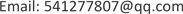1辽宁工程技术大学，辽宁省高等学校矿产资源开发利用技术及装备研究院，辽宁 阜新

2辽宁工程技术大学，机械工程学院，辽宁 阜新

3山西煤炭进出口集团鹿台山煤业有限公司，山西 晋城1. 引言

2. 运动学模型建立

R P Y ( β , γ , α ) = R o t ( y , γ ) R o t ( x , β ) = [ cos α cos γ − sin α cos β + cos α sin γ sin β sin α sin β + cos α sin γ cos β sin α cos γ cos α cos β + sin α sin γ sin β − cos α sin β + sin α sin γ cos β − sin γ cos sin β cos γ cos β ] (2.1)

T 0 = [ cos α cos γ − sin α cos β + cos α sin γ sin β sin α sin β + cos α sin γ cos β a sin α cos γ cos α cos β + sin α sin γ sin β − cos α sin β + sin α sin γ cos β b − sin γ cos γ sin β cos γ cos β c 0 0 0 0 ] (2.2)

γ ——机身绕y轴旋转角；

α ——机身绕z轴旋转角；

( a , b , c ) ——坐标系 O 1 - X 1 Y 1 Z 1 的原点在 O 0 - X 0 Y 0 Z 0 中的坐标。

p 0 = T 0 p 1 = [ cos α cos γ L x + ( − sin α cos β + cos α sin γ sin β ) L y + a sin α cos γ L x + ( cos α cos β + sin α sin γ sin β ) L y + b − sin γ L x + cos γ sin β L y + c 1 ] (2.3)

p 0 = [ cos α L x − sin α L y + a sin α L x + cos α L y + b c 1 ] (2.4)

3. 转向运动关系

ω ( R + ( − 1 ) i B / 2 ) + ω A i = v i ,   i = 1 , 2 (3.1)

ω ——车辆转动的角速度；

R 1 ——理论转向半径。

A i = v a y i / ω ,   i = 1 , 2 (3.2)

{ a i = A i / ( L / 2 ) d = D / ( L / 2 ) (3.3)

ρ = 1 2 ( 1 + λ a 1 ) + K v ( 1 + λ a 2 ) 1 − K v = f ρ ρ t (3.4)

ρ t ——不考虑履带滑移情况的相对转弯半径， ρ t = 1 2 ( 1 + K v 1 − K v ) ；

λ ——履带贴地长度L和履带中心距离B之比， λ = L / B ；

fρ——履带滑移时，相对转弯半径和不考虑履带滑移的相对转弯半径比例系数，即转弯半径修正系数，表达式为：

f ρ = ( 1 + λ a 1 ) + K v ( 1 + λ a 2 ) 1 + K v (3.5)

ω = v 2 − v 1 B ′ + A 2 + A 1 = f ω ω t (3.6)

ω t ——不考虑履带滑移条件下的角速度， ω t = ( v 2 − v 1 ) / B ′ ；

f ω ——考虑履带滑移时相对转弯半径和不考虑履带滑移的角速度比例系数，即转弯半径修正系数，表达式为：

f ω = 1 1 + λ / 2 ( a 2 − a 1 ) (3.7)

{ v r = ( ρ − 1 2 B ) ω v l = ( ρ + 1 2 B ) ω (3.8)

{ v r = R ω r v l = R ω l (3.9)

ω = R ω r ρ − 1 2 B = R ω L ρ + 1 2 B (3.10)

ω = R ( ω l − ω r ) B (3.11)

ρ = 1 2 B ( ω l + ω r ) ω l − ω r = 1 2 B ( 1 + 2 ω l / ω r − 1 ) (3.12)

4. 防爆履带式液压支架搬运车越障运动分析

{ { T + G ( L x cos α − L y sin α ) + F f B cos α ( R + L sin α − R ) − ( F f B sin α + F N B ) L 1 cos α = 0 F f B = μ F N B (4.1)

F N A , F N B ——搬运车受到地面的支撑力；

G——搬运车本体结构重力；

T——输出转矩；

μ ——摩擦系数，取 μ = 0.5 ；

α ——搬运车仰角，其中规定防爆履带式液压支架搬运车逆时针旋转为正。

T = ( F f B sin α + F N B ) L 1 cos α − G ( L x cos α − L y sin α ) − F f B cos α L sin α (4.2)

5. 结论